# mokacoding

unit and acceptance testing, automation, productivity

## Swift Functors, Applicatives, and Monads in Pictures

This is a translation of Functors, Applicatives, And Monads In Pictures from Haskell into Swift.

I don't want to take any merit for writing this, I only went through the fun exercise of translating the code snippets in Swift.

If you enjoy this post be sure to say thanks to the author of the original version: Aditya Bhargava, @_egonschiele on Twitter.

Despite all the hype about it, Swift is not a functional language. This means that we need to write a bit of extra code to achieve the same results that language like Haskell have with built-in operators.

You can find a Playground with all the code from the article on GitHub.

Finally, don't worry if you find the content hard to grasp. I had to read the original version a number of times to wrap my head around it, plus a lot of mess around with the Swift code.

Here’s a simple value: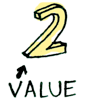And we know how to apply a function to this value:Simple enough. Lets extend this by saying that any value can be in a context. For now you can think of a context as a box that you can put a value in: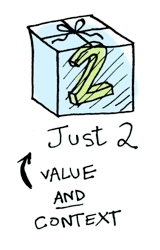Now when you apply a function to this value, you’ll get different results depending on the context. This is the idea that Functors, Applicatives, Monads, Arrows etc are all based on. The `Optional` type defines two related contexts:

Note: the pictures use Maybe (Just | None) from Haskell, which correspond to Swift's Optional .Some and .None.``````enum Optional<T> {
case None
case Some(T)
}``````

In a second we will see how function application is different when something is a `.Some(T)` versus a `.None`. First let’s talk about Functors!

# Functors

When a value is wrapped in a context, you can’t apply a normal function to it: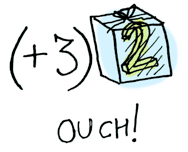This is where `map` comes in (`fmap` in Haskell). `map` is from the street, `map` is hip to contexts. `map` knows how to apply functions to values that are wrapped in a context. For example, suppose you want to apply a function that adds 3 to `.Some(2)`. Use `map`:

``````func plusThree(addend: Int) -> Int {
}

Optional.Some(2).map(plusThree)
// => .Some(5)``````

or with a simple syntax using Swift's autoclosure:

``````Optional.Some(2).map { \$0 + 3 }
// => .Some(5)``````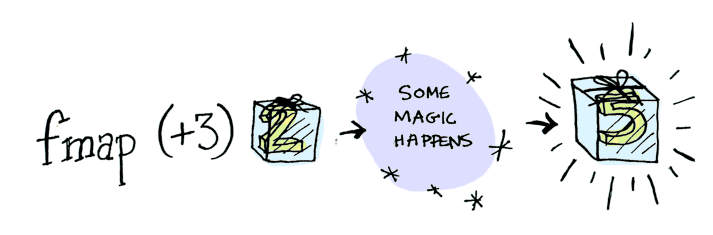Bam! `map` shows us how it’s done! But how does `map` know how to apply the function?

# Just what is a Functor, really?

A Functor is any type that defines how `map` (`fmap` in Haskell) applies to it. Here’s how `map` works: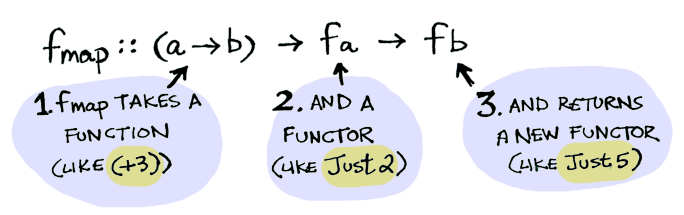So we can do this:

``````Optional.Some(2).map { \$0 + 3 }
// => .Some(5)``````

And `map` magically applies this function, because `Optional` is a Functor. It specifies how `map` applies to `Some`s and `None`s:

``````func map<U>(f: T -> U) -> U? {
switch self {
case .Some(let x): return f(x)
case .None: return .None
}``````

Here’s what is happening behind the scenes when we write `Optional.Some(2).map { \$0 + 3 }`: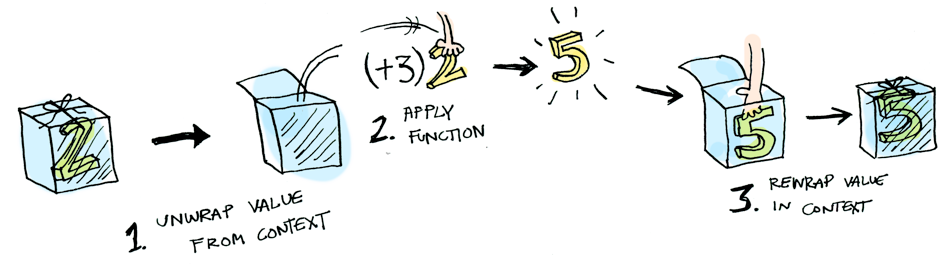So then you’re like, alright `map`, please apply `{ \$0 + 3 }` to a `.None`?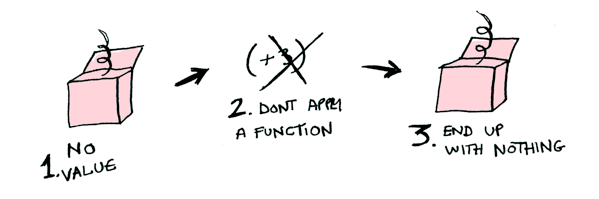``````Optional.None.map { \$0 + 3 }
// => .None``````Bill O’Reilly being totally ignorant about the Maybe functor

Like Morpheus in the Matrix, `map` knows just what to do; you start with `None`, and you end up with `None`! `map` is zen. Now it makes sense why the `Optional` type exists. For example, here’s how you work with a database record in a language without `Optional`, like Ruby:

``````let post = Post.findByID(1)
if post != nil {
return post.title
} else {
return nil
}``````

But in with Swift using the `Optional` functor:

``findPost(1).map(getPostTitle)``

If `findPost(1)` returns a post, we will get the title with `getPostTitle`. If it returns `None`, we will return `None`!

We can even define an infix operator for `map`, `<^>` (`<\$>` in Haskell), and do this instead:

``````infix operator <^> { associativity left }

func <^><T, U>(f: T -> U, a: T?) -> U? {
return a.map(f)
}

getPostTitle <^> findPost(1)``````

Note: we have to use `<^>` because `<\$>` wouldn't compile.

Here’s another example: what happens when you apply a function to an array?Arrays are functors too!

Okay, okay, one last example: what happens when you apply a function to another function?

``````map({ \$0 + 2 }, { \$0 + 3 })
// => ???``````

Here's a function: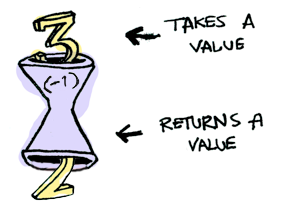Here’s a function applied to another function: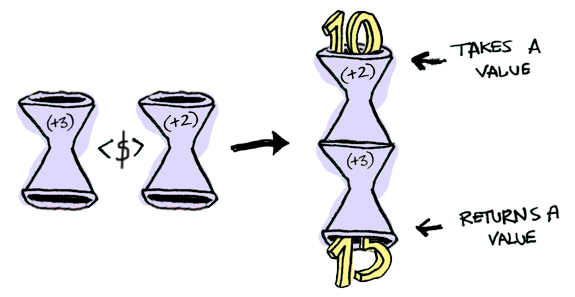The result is just another function!

``````typealias IntFunction = Int -> Int

func map(f: IntFunction, _ g: IntFunction) -> IntFunction {
return { x in f(g(x)) }
}

let foo = map({ \$0 + 2 }, { \$0 + 3 })
foo(10)
// => 15``````

So functions are Functors too! When you use fmap on a function, you’re just doing function composition!

# Applicatives

Applicatives take it to the next level. With an applicative, our values are wrapped in a context, just like Functors:But our functions are wrapped in a context too!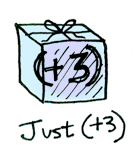Yeah. Let that sink in. Applicatives don’t kid around. Unlike Haskell, Swift doesn't have yet a built-in way to deal with Applicative. But it is very easy to add one! We can define an `apply` function for every type supporting Applicative, which knows how to apply a function wrapped in the context of the type to a value wrapped in the same context:

``````extension Optional {
func apply<U>(f: (T -> U)?) -> U? {
switch f {
case .Some(let someF): return self.map(someF)
case .None: return .None
}
}
}

extension Array {
func apply<U>(fs: [Element -> U]) -> [U] {
var result = [U]()
for f in fs {
for element in self.map(f) {
result.append(element)
}
}
return result
}
}``````

If both `self` and the function are `.Some`, then the function is applied to the unwrapped option, otherwise `.None` is returned. Also note that because the optional type is defined in terms of `Optional<T>` we only need to specify the generic type `U` in `apply`s signature.

We can also define `<*>`, to do the same thing:

``````infix operator <*> { associativity left }

func <*><T, U>(f: (T -> U)?, a: T?) -> U? {
return a.apply(f)
}

func <*><T, U>(f: [T -> U], a: [T]) -> [U] {
return a.apply(f)
}``````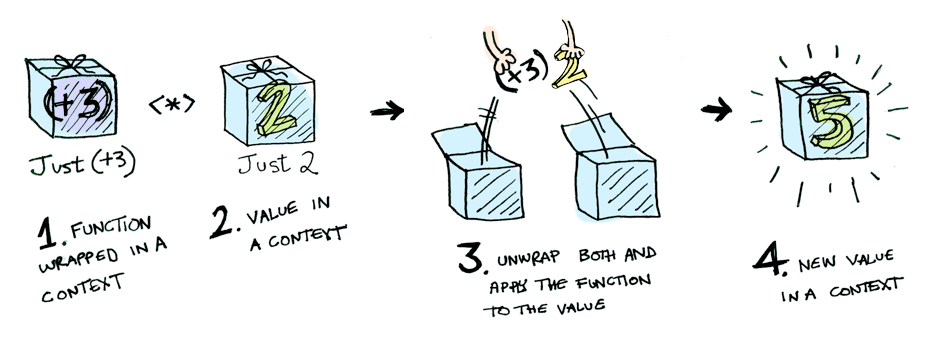i.e:

``````Optional.Some({ \$0 + 3 }) <*> Optional.Some(2)
// => 5``````

Using `<*>` can lead to some interesting situations. For example:

``````
[ { \$0 + 3 }, { \$0 * 2 } ] <*> [1, 2, 3]
// => [ 4, 5, 6, 2, 4, 6 ]``````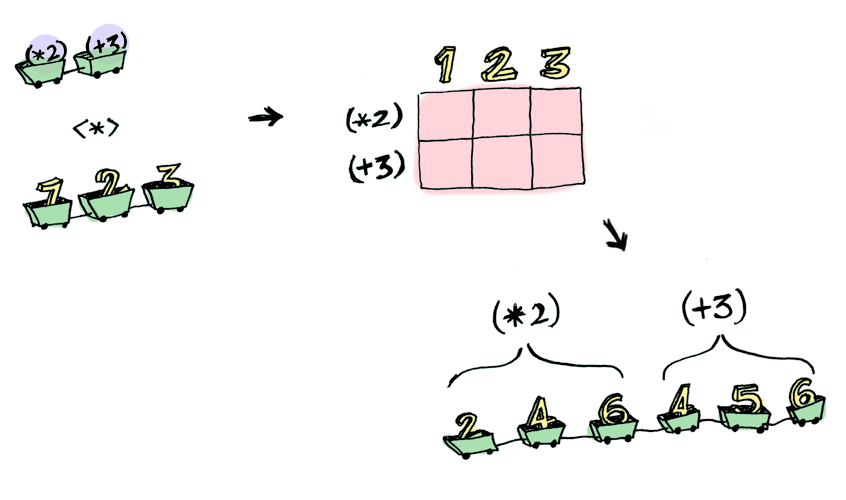Note: the original article now shows how Applicatives are more powerful than Functors in that they allow function application with multiple parameters. Again this is not feasible in vanilla Swift, but we can work around it by defining the function we want to handle in a curried way.

Here’s something you can do with Applicatives that you can’t do with Functors. How do you apply a function that takes two arguments to two wrapped values?

``````func curriedAddition(a: Int)(b: Int) -> Int {
return a + b
}

// => COMPILER ERROR: Value of optional type '(Int -> Int)? not unwrapped; did you mean to use '!' or '??'``````

Applicatives:

``curriedAddition <^> Optional(2) <*> Optional(3)``

`Applicative` pushes `Functor` aside. “Big boys can use functions with any number of arguments,” it says. “Armed with `<^>` and `<*>`, I can take any function that expects any number of unwrapped values. Then I pass it all wrapped values, and I get a wrapped value out! AHAHAHAHAH!”

``````func curriedTimes(a: Int)(b: Int) -> Int {
return a * b
}

curriedTimes <^> Optional(5) <*> Optional(3)``````

1. Get a PhD in computer science.
2. Throw it away because you don’t need it for this section!

Functors apply a function to a wrapped value: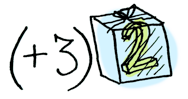Applicatives apply a wrapped function to a wrapped value: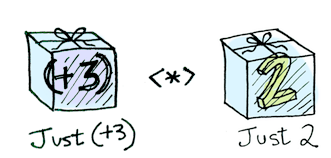Monads apply a function that returns a wrapped value to a wrapped value. Monads have a function `|` (>>= in Haskell) (pronounced “bind”) to do this.

Monads have a function `flatMap` (`liftM` in Haskell) to do this. And we can define an infix operator `>>-` (`>>=` in Haskell) for it.

``````infix operator >>- { associativity left }

func >>-<T, U>(a: T?, f: T -> U?) -> U? {
return a.flatMap(f)
}``````

Note: Unlike `<\$>`, `>>=` would compile. I decided to use `>>-` to be in line with the library Runes which provides "Infix operators for monadic functions in Swift", and it's hopefully going to become the standard for this sort of things.

Let’s see an example. Good ol’ Optional is a monad:Suppose `half` is a function that only works on even numbers:

``````func half(a: Int) -> Int? {
return a % 2 == 0 ? a / 2 : .None
}``````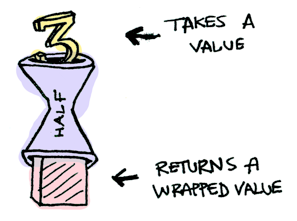What if we feed it a wrapped value?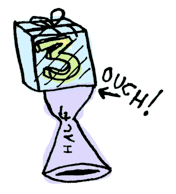We need to use `>>-` (`>>=` in Haskell) to shove our wrapped value into the function. Here’s a photo of `>>-`:Here’s how it works:

``````Optional(3) >>- half
// .None
Optional(4) >>- half
// 2
Optional.None >>- half
// .None``````

What's happening inside? Let's look at `>>-`'s (`>>=` in Haskell) signature again:

``````// For Optional
func >>-<T, U>(a: T?, f: T -> U?) -> U?

// For Array
func >>-<T, U>(a: [T], f: T -> [U]) -> [U]``````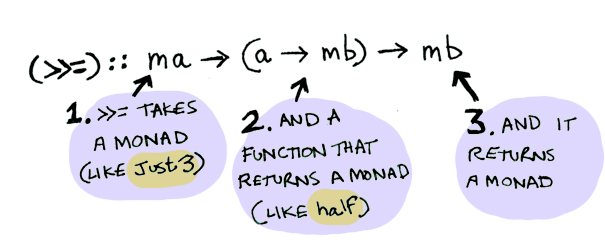So `Optional` is a Monad. Here it is in action with a `.Some(3)`!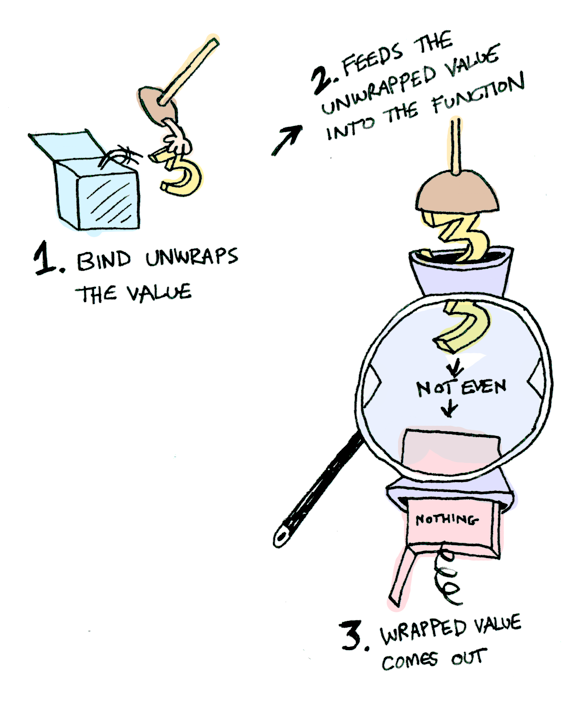And if you pass in a `.None` it’s even simpler: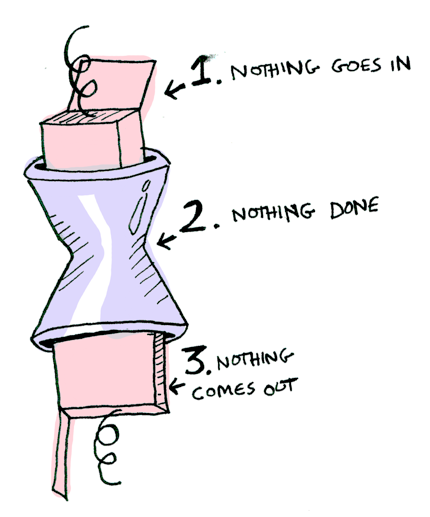You can also chain these calls:

``````Optional(20) >>- half >>- half >>- half
// => .None``````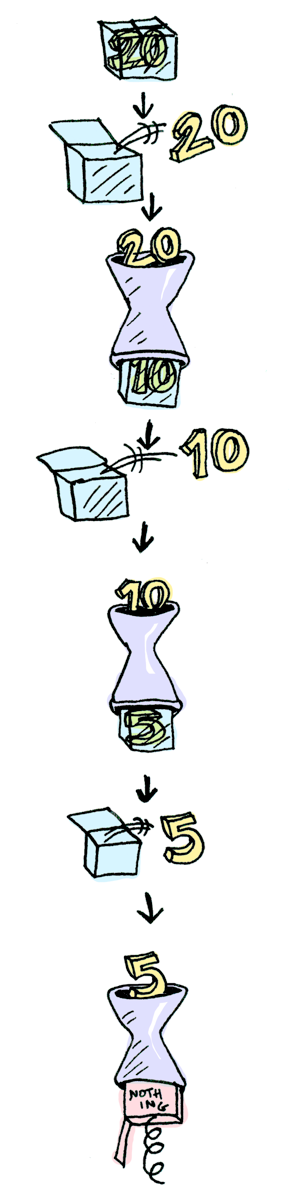NOte: the original article now describes Haskell's `IO` Monad. Swift doesn't have anything like that so this translation skips it.

# Conclusion

1. A functor is a type that implements `map`.
2. An applicative is a type that implements `apply`.
3. A monad is a type that implements `flatMap`.
4. `Optional` implements `map` and `flatMap`, plus we can extend it to implement `apply`, so it is a functor, an applicative, and a monad.

What is the difference between the three?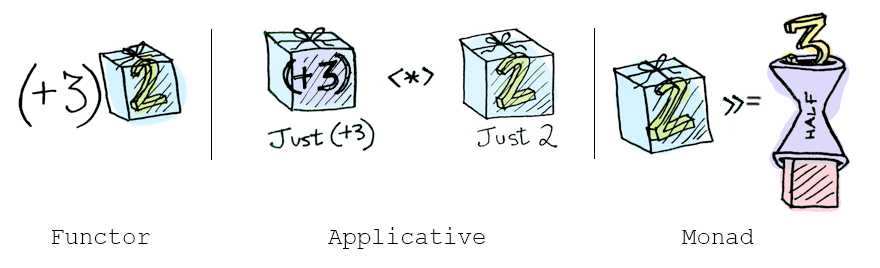• functors: you apply a function to a wrapped value using `map`.
• applicatives: you apply a wrapped function to a wrapped value using `apply`, if defined.
• monads: you apply a function that returns a wrapped value, to a wrapped value using `flatMap`.

So, dear friend (I think we are friends by this point), I think we both agree that monads are easy and a SMART IDEA(tm). Now that you’ve wet your whistle on this guide, why not pull a Mel Gibson and grab the whole bottle. Check out LYAH’s section on Monads. There’s a lot of things I’ve glossed over because Miran does a great job going in-depth with this stuff.